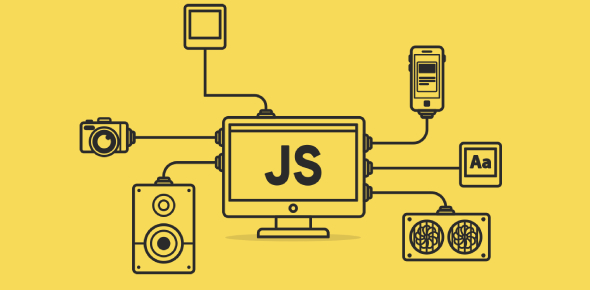# JavaScript Exam: MCQ Quiz!

25 Questions | Total Attempts: 77Settings.

• 1.
Do functions in JavaScript necessarily return a value?
• A.

It is mandatory

• B.

Not necessary

• C.

Few functions return values by default

• D.

All of the choices

• 2.
Which of the following is an example of a javascript function?
• A.

• B.

If()

• C.

Script()

• D.

None of the choices

• 3.
It is a group of reusable code which can be called anywhere in your program.
• A.

Events

• B.

Functions

• C.

Actions

• D.

Objects

• 4.
Consider the following code snippet: var tensquared = (function(x) {return x*x;}(10)); Will the given code work?
• A.

Yes, perfectly

• B.

Error

• C.

Exception will be thrown

• D.

Memory leak

• 5.
Consider the following code snippet.
• A.

123

• B.

123xyz

• C.

Exception

• D.

NaN

• 6.
For the below-mentioned code snippet: var o = new Object(); The equivalent statement is
• A.

Var o = Object();

• B.

Var o;

• C.

Var o= new Object;

• D.

Object o=new Object();

• 7.
The most common way to define a function in JavaScript is by using the________ keyword.
• A.

Event

• B.

Func

• C.

Function

• D.

Script

• 8.
What is the output of the following snippet: <html> <head> <script type="text/javascript"> function sayHello() { document.write ("Hello there!"); } </script> </head> <body> <p>Click the following button to call the function</p> <form> <input type="button" onclick="sayHello()" value="Say Hello"> </form> <p>Use different text in write method and then try...</p> </body> </html>
• A.

When the button is clicked an output sayHell will be displayed Option 1

• B.

When the button is clicked an output "Hello" will be displayed

• C.

When the button is clicked an output "Button" will be displayed

• D.

When the button is clicked an output "Hello there!" will be displayed

• 9.
Which of the following statement about the function is true?
• A.

Functions would not eliminates the need of writing the same code again and again

• B.

A function can take multiple parameters separated by comma.

• C.

It will not allow a programmer to divide a big program into a number of small and manageable functions

• D.

The parameter list of the function should be empty

• 10.
The follwing syntax is used to?<script type="text/javascript"><!--var variablename = new Function(Arg1, Arg2..., "Function Body");//--></script>
• A.

To create a function using Function() constructor

• B.

To implement nested functions.

• C.

To implement function literal

• D.

None of the choices

• 11.
Consider the following code snippet: function constfuncs() { var funcs = []; for(var i = 0; i < 10; i++) funcs[i] = function() { return i; }; return funcs; } var funcs = constfuncs(); funcs() What does the last statement return?
• A.

9

• B.

0

• C.

10

• D.

None of the choices

• 12.
The behavior of the instances present of a class inside a method is defined by:
• A.

Method

• B.

Classes

• C.

Interfaces

• D.

Classes and Interfaces

• 13.
The keyword or the property that you use to refer to an object through which they were invoked is:
• A.

From

• B.

To

• C.

This

• D.

Object

• 14.
JavaScript's interaction with HTML is handled through ___ that occurs when the user or the browser manipulates a page.
• A.

Function

• B.

Class

• C.

Object

• D.

Event

• 15.
This is the most frequently used event type which occurs when a user clicks the left button of his mouse.
• A.

OnSubmit

• B.

Onmouseover

• C.

OnClick

• D.

All of the given

• 16.
It is is an important keyword in JavaScript which can be used as a unary operator that appears before its single operand, which may be of any type.
• A.

Return

• B.

Void

• C.

Function

• D.

Script

• 17.
It is the capability to store related information, whether data or methods, together in an object.
• A.

Encapsulation

• B.

Aggregation

• C.

Inheritance

• D.

Polymorphism

• 18.
It is the capability to store one object inside another object.
• A.

Polymorphism

• B.

Encapsulation

• C.

Inheritance

• D.

Aggregation

• 19.
It is the capability to write one function or method that works in a variety of different ways.
• A.

Polymorphism

• B.

Encapsulation

• C.

Inheritance

• D.

Aggregation

• 20.
It is the capability of a class to rely upon another class (or number of classes) for some of its properties and methods.
• A.

Encapsulation

• B.

Inheritance

• C.

Aggregation

• D.

Polymorphism

• 21.
The syntax for adding a property to an object is:
• A.

ObjectName.objectProperty = new propertyValue();

• B.

ObjectName.objectProperty = new propertyValue;

• C.

ObjectName.objectProperty = propertyValue;

• D.

ObjectName.objectProperty = propertyValue();

• 22.
Which of the following is an example to show how to use the write() method of the document object to write any content on the document?
• A.

• B.

Document.write ("Hello world");

• C.

Document.write (This is test);

• D.

None of the given

• 23.
The____ operator is used to create an instance of an object.
• A.

New

• B.

This

• C.

Void

• D.

• 24.
A _______is a function that creates and initializes an object.
• A.

Constructor

• B.

Object

• C.

Events

• D.

Var

• 25.
Which of the following syntax is an example of properly declaring a function?
• A.

Fun sayHello();

• B.

Func say Hello()

• C.

Book name is : Perl Book name is : Perl function sayHello()

• D.

Function sayHello;

Related TopicsBack to top